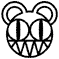Mathematics

Whoops! did you mean Maths?

Do the math. Don't worry, it's just a math-er of time before you get it.

$Math/Sc^{(ho)}xol=teh^{suck}$$Banana/(4*QuantumSnack)=Math$$Koala'd=SuperJapan$$Bandicoot'd=UltraJapan$$Subbutians=Fullof/THEMSELVES$$URMOM+HAW-T=LOLZ!!$$Me^{Movement}=Street*Crime$$Math/(4+/32|0)/FUCK=nerdporn$This page has received the blessing of The Great Gozimnot of Mathematics, and is therefore mathematically correct.